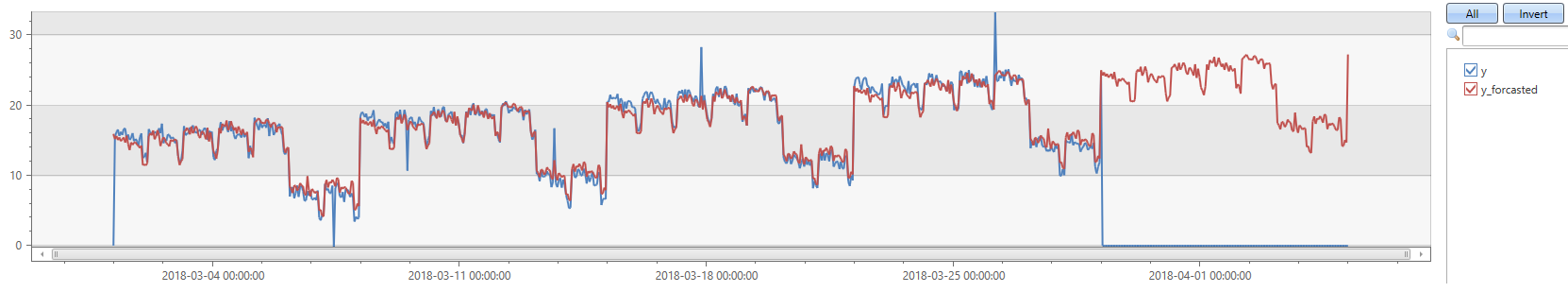# series_decompose_forecast()

Forecast based on series decomposition.

Takes an expression containing a series (dynamic numerical array) as input, and predicts the values of the last trailing points. For more information, see series_decompose.

## Syntax

`series_decompose_forecast(`Series`,` Points`,` [ Seasonality`,` Trend`,` Seasonality_threshold ]`)`

## Parameters

Name Type Required Description
Series dynamic An array of numeric values, typically the resulting output of make-series or make_list operators.
Points int Specifies the number of points at the end of the series to predict, or forecast. These points are excluded from the learning, or regression, process.
Seasonality int Controls the seasonal analysis. The possible values are:

- `-1`: Autodetect seasonality using series_periods_detect. This is the default value.
- Period: A positive integer specifying the expected period in number of bins. For example, if the series is in `1 - h` bins, a weekly period is 168 bins.
- `0`: No seasonality, so skip extracting this component.
Trend string Controls the trend analysis. The possible values are:

- `avg`: Define trend component as `average(x)`. This is the default.
- `linefit`: Extract trend component using linear regression.
- `none`: No trend, so skip extracting this component.
Seasonality_threshold real The threshold for seasonality score when Seasonality is set to autodetect. The default score threshold is 0.6.

## Returns

A dynamic array with the forecasted series.

Note

• The dynamic array of the original input series should include a number of points slots to be forecasted. The forecast is typically done by using make-series and specifying the end time in the range that includes the timeframe to forecast.
• Either seasonality or trend should be enabled, otherwise the function is redundant, and just returns a series filled with zeroes.

## Example

In the following example, we generate a series of four weeks in an hourly grain, with weekly seasonality and a small upward trend. We then use `make-series` and add another empty week to the series. `series_decompose_forecast` is called with a week (24*7 points), and it automatically detects the seasonality and trend, and generates a forecast of the entire five-week period.

``````let ts=range t from 1 to 24*7*4 step 1 // generate 4 weeks of hourly data
| extend Timestamp = datetime(2018-03-01 05:00) + 1h * t
| extend y = 2*rand() + iff((t/24)%7>=5, 5.0, 15.0) - (((t%24)/10)*((t%24)/10)) + t/72.0 // generate a series with weekly seasonality and ongoing trend
| extend y=iff(t==150 or t==200 or t==780, y-8.0, y) // add some dip outliers
| extend y=iff(t==300 or t==400 or t==600, y+8.0, y) // add some spike outliers
| make-series y=max(y) on Timestamp from datetime(2018-03-01 05:00) to datetime(2018-03-01 05:00)+24*7*5h step 1h; // create a time series of 5 weeks (last week is empty)
ts
| extend y_forcasted = series_decompose_forecast(y, 24*7)  // forecast a week forward
| render timechart
``````